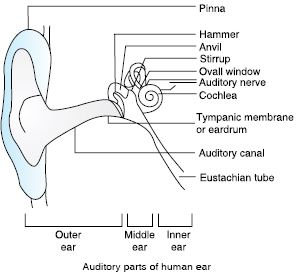# NCERT Solutions for Class 9 Science Chapter 12 - Sound

##### Question 1:

How does the sound produced by a vibrating object in a medium reach your ear?

Sound in the air gets propagated in the form of longitudinal wave motion consisting of the regions of compressions and rarefactions. Consider, for example, a tuning fork in a state of vibration [Fig. 1(a)]. As a prong moves towards the right, it compresses the layer of air in contact with it. As air has elasticity, the compressed air tends to relieve itself of its strain and moves forward to the right to compress the next layer and so on. Thus, a wave of compression moves towards the right. At the point of compression, there is an increase of pressure (shown by C). At the point of rarefaction, the concentration of particles is least (shown by R).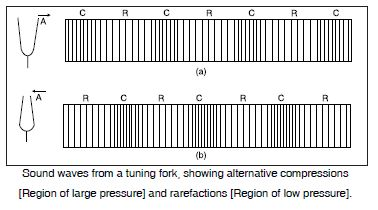When the prong moves towards left, a region of reduced pressure or rarefaction is produced towards the right [Fig. 1(b)]. The air in the next layer, being at a higher pressure, move towards left to equalise the reduced pressure producing another rarefied region to its right and so on.
Thus, a wave of rarefaction starts moving towards right. In this way, a series of compressions and rarefactions move in the forward direction. These compressions and rarefactions finally reach the ear-drum of the observer and we can hear sound.

##### Question 2:

Explain how the sound is produced by your school bell.

When the school bell is struck by a hammer, it starts moving forward and backward due to elasticity and inertia. During the forward motion of the bell, a wave of increased pressure i.e., compression is produced. When the bell moves backward, a wave of rarefaction i.e., a wave of decreased pressure is produced. When a series of compressions and rarefactions reach our ear, it results in the production of sound in our ear.

##### Question 3:

Why are sound waves called mechanical waves?

Sound waves are called mechanical waves because these are produced due to the forward and backward motion of the material particles. Sound cannot travel in vacuum. A material medium is essential for the production of sound waves.

##### Question 4:

Suppose you and your friend are on the moon. Will you be able to hear any sound produced by your friend?

No, you will not be able to hear any sound when you and your friend are on moon. This is because there is no atmosphere on the moon. For transmission of sound, a material medium is must.

##### Question 5:

Which wave property determines (a) loudness (b) pitch?

(a) Amplitude determines loudness. The greater the amplitude, the greater is the loudness. It also depends upon the square of the amplitude of wave. Loudness also depends upon intensity of sound as well as sensitivity of ear.
(b) Pitch depends upon the frequency of sound. The more the frequency, the higher is the pitch of sound. Shrillness of sound also depends upon its pitch. The more the pitch, the shriller is the sound.

##### Question 6:

Guess which sound has a higher pitch: guitar or a car horn.

Though car horn is louder than guitar, the latter has a higher pitch than car horn. Therefore, the frequency of guitar is higher than that of car horn.

##### Question 7:

What are wavelength, frequency, time period and amplitude of a sound wave?

Wavelength is the distance between two consecutive compressions or two consecutive rarefactions.
It is usually represented by l and its SI unit is metre.
Frequency is the number of oscillations completed in a unit time.
It is represented by v and its SI unit is s-1 or Hertz (Hz).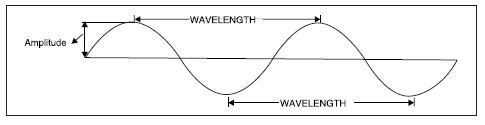Time period is the time taken to complete one oscillation. It is represented by T and its SI unit is second.
T = 1/v or v = 1/T
Amplitude is the maximum displacement of the particles of the medium on either side of the mean position. It is usually represented by A and its SI unit is metre.

##### Question 8:

How are the wavelength and frequency of a sound wave related to its speed?

The relation is v = vl, where v is the speed, v is the frequency and l is the wavelength.

##### Question 9:

Calculate the wavelength of a sound wave whose frequency is 220 Hz and speed is 440 ms-1 in a given medium.

Here v = 220 Hz; v = 440 ms-1; l = ?
v = v l

l =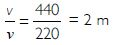##### Question 10:

A person is listening to a tone of 500 Hz sitting at a distance of 450 m from the source of sound. What is the time interval between successive compressions from the source?

Here v = 500 Hz; T = ?
[Time between successive compressions is the time period].
T = 1/v = 1/ (500)
T = 0.002 s.

##### Question 11:

Distinguish between loudness and intensity of sound.

Loudness of sound is a measure of response of the ear to the sound. It depends upon the intensity of sound (sound energy per unit area per unit time) and the sensitivity of the ear. Two sounds of equal intensity falling upon the ears of two persons may appear to be having different loudness. However, the more the intensity, the louder is the sound.
Therefore, besides sensitiveness of the ear, the loudness of sound depends upon all the factors on which intensity of sound depends. It also depends upon the density of air. The more the density, the louder is the sound.

##### Question 12:

In which of the three media, air, water or iron, does sound travel the fastest at a particular temperature?

It travels fastest in iron [about 5,500 ms-1], followed by water [about 1,500 ms-1]. It travels slowest in air [about 340 ms-1] at a given temperature.

##### Question 13:

An echo was heard after 3 s. What is the distance of the reflecting surface from the source, given that the speed of sound is 342 ms-1?

Let the distance of reflecting surface from the source be x metres. In going and coming back after reflection, the sound has travelled 2 x m.
s = 2 x m; v = 342 m s-1; t = 3 s
s = vt
2x = 342 x 3 = 1,026
x = 513 m

##### Question 14:

Why are the ceilings of concert halls curved?

Ceilings of concert halls, conference halls etc. are curved so that sound reaches all corners of the hall after multiple reflections. Sometimes curved sound board is placed behind the stage so that after reflection from the sound board, the sound spreads evenly across the width of the hall.

##### Question 15:

What is the audible range of average human ear?

An average human ear can hear frequencies between 20 Hz to 20,000 Hz.

##### Question 16:

What is the range of frequencies associated with (a) infrasound (b) ultrasound?

(a) Infrasound is a sound of frequency below 20 Hz. Rhinoceroses communicate with each other using frequency as low as 5 Hz. Elephants and whales also produce infrasound.
(b) Ultrasonics or ultrasounds are sounds of frequency higher than 20 kHz [20,000 Hz]. Ultrasounds are produced by dolphins, bats, rats and porpoises. Moths can hear ultrasounds and detect ultrasounds produced by bats.

##### Question 17:

A submarine emits a sonar pulse, which returns from an underwater cliff in 1.02 s. If the speed of sound in saltwater is 1,531 ms-1, how far away is the cliff?

Let the cliff be at a distance of x metres. Distance travelled by sound in going and coming back = 2x metres.
Time taken, t = 1.02 s; v = 1,531 ms-1.
s = v t
2x = 1,531 × 1.02
x = 1,531 × 0.51
= 780.81 m

##### Question 18:

What is sound and how is it produced?

Sound is a form of energy which produces a sensation of hearing in our ears.
It is produced due to the vibrations of a body about its mean position. For example, a bell and wires in a sitar produce sound on vibrating.

##### Question 19:

Describe with the help of a diagram, how compressions and rarefactions are produced in the air near a source of sound.

Sound in air gets propagated in the form of longitudinal wave consisting of regions of compressions and rarefactions. Consider a tuning fork in a state of vibration [Fig. (a)]. As a prong moves towards right, it compresses the layer of air in contact with it. As air has elasticity, the compressed air tends to relieve itself of its strain and moves forward to the right to compress the next layer and so on. Thus, a wave of compression moves towards the right. At the point of compression, there is an increase of pressure and is shown in the form of crest C. At the point of rarefaction, the concentration of particles is least and has been shown as trough R.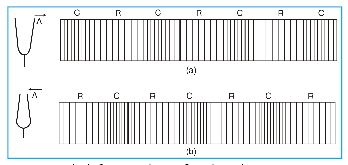When the prong moves towards left, a region of reduced pressure or rarefaction is produced towards right [Fig. (b)]. The air in the next layer, being at a higher pressure, moves towards the left to equalise the reduced pressure producing another rarefied region to its right and so on. In this way a series of compressions and rarefactions move in forward direction. These compressions and rarefactions finally reach the ear drum of the observer and we hear sound.

##### Question 20:

Cite an experiment to show that sound needs a material medium for its propagation.

It can be shown that a material medium is essential for the propagation of sound waves with the help of the bell jar experiment.
Procedure:
i. Take an inverted glass jar V and place it on a vacuum pump. Fit an electric bell in the jar as shown.
ii. Take the air out of the glass jar with the help of a vacuum pump.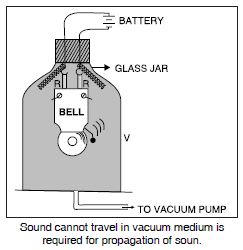Observation:
When air is steadily taken out of the glass jar, the sound of the bell goes on becoming fainter and fainter till it is inaudible. However, vibrations of gong of the bell can be seen from outside.
Conclusion:
i. A material medium is required by the sound for its propagation.
ii. Sound cannot travel in vacuum.

##### Question 21:

Why is a sound wave called a longitudinal wave?

Longitudinal wave motion is defined as a motion in which the individual particles of the medium vibrate back and forth along the direction of propagation of the wave.
When sound moves in a medium, each particle of the medium vibrates about its mean position in the direction of wave propagation. That is why sound waves are called longitudinal waves.

##### Question 22:

Which characteristic of sound helps you to identify your friend by his voice while sitting with others in a dark room?

Quality (or timbre) and pitch of sound are the characteristics which enable us to distinguish one sound from another even in the dark room.

##### Question 23:

Flash and thunders are produced simultaneously. But thunder is heard a few seconds after the flash is seen. Why?

The speed of sound is about 340 ms-1. However, the speed of light is extremely large i.e., 3 × 105 kms-1 [3,00,000 km/s]. The flash of light between clouds reaches almost immediately and can be seen earlier than hearing the sounds of thunder. That’s why thunder is heard a few seconds after the flash of thunder is seen instead of both are produced simultaneously.

##### Question 24:

A person has a hearing range from 20 Hz to 20 kHz. What is the typical wavelength of sound waves in air corresponding to these frequencies? Take the speed of sound in air as 344 ms-1.

(i) v = 344 ms-1; v = 20 Hz; l = ?
v = v l

or l =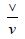l =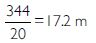(ii) v = 20 kHz = 20,000 Hz; l = ?; v = 344 ms-1

l =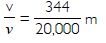l = 17.2 × 10-3 m = 17.2 mm

##### Question 25:

Two children are at opposite ends of an aluminium rod. One strikes the end of the rod with a stone. Find the ratio of times taken by the sound waves in air and in aluminium to reach the second child.

Let l be the length of the rod. Let vair and val be the velocities of sound in air and aluminium respectively.
vair = 346 m s-1; val = 6,420 m s-1
Let d be the distance travelled by the wave.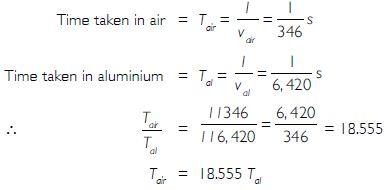##### Question 26:

The frequency of a source of sound is 100 Hz. How many times does it vibrate in a minute?

Vibrations made in 1 second = Frequency = 100
Vibrations made in 1 minute or in 60 s = 100 × 60
= 6,000

##### Question 27:

Does sound follow the same laws of reflection as light does? Explain.

Yes, sound follows the same laws of reflection as light i.e.,
1. Incident wave, reflected wave and normal all lie in the same plane.
2. The angle of incidence is equal to the angle of reflection.
Verification:
To verify the laws of reflection, take two tubes A and B about 1 m long and 5 to 7 cm in diameter. Mount the tubes as shown in the figure. Place a watch at the end of the tube A and try to hear the sound with your ear at the end of the tube B. Place a screen S made of cardboard in between the two tubes to prevent sound from watch to reach ear directly. It will be observed that the sound heard by your ear will be maximum when angles made by A and B with normal are equal i.e., angle of incidence (∠i) = angle of reflection (∠r).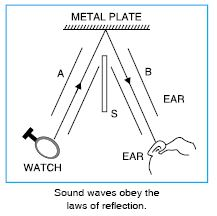##### Question 28:

When a sound is reflected from a distant object, an echo is produced. Let the distance between reflected surface and the source of sound production remains the same. Do you hear echo sound on a hotter day?

The speed of sound in air increases by about 0.6 ms-1 for every 1°C rise in temperature. Echo is heard till the reflected sound reaches our ear after 1/(10) = 0.1 s. As the temperature is increased, the reflected sound takes less and lesser time to retrace back. When the temperature increases to such an extent that reflected sound takes less than 1/(10) s to return, no echo will be heard.

##### Question 29:

Give two practical applications of reflection of sound waves.

i. Stethoscope is used for listening to heartbeats produced by multiple reflections of sound.
ii. Megaphones or loudspeakers, musical instruments like shehnais are designed to send sound in a particular direction without spreading them in all directions.

##### Question 30:

A stone is dropped from the top of a tower 500 m high into a pond of water at the base of the tower. When is the splash heard at the top? Given, g = 10 ms-2 and speed of sound is 340 ms-1.

Let t be the time taken by stone to cover 500 m downwards.
u = 0; a = 10 ms-2; s = 500 m; t = ?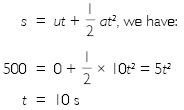Let t′ be time taken by the sound to reach the top after splash.
u = 340 ms-1; s = 500 m; t′= ?
Sound travels with uniform speed, \ a = 0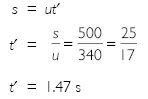\ Total time to hear the sound of splash = t + t′= 10 + 1.47 = 11.47 s.

##### Question 31:

A sound wave travels at a speed of 339 ms-1. If its wavelength is 1.5 cm, what is the frequency of the wave? Will it be audible?

v = 339 ms-1; l = 1.5 cm = 0.015 m; v = ?
v = vl or v = v/l

v =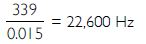Since it is greater than 20,000 Hz, it will not be audible to human beings.

##### Question 32:

What is reverberation? How can it be reduced?

A sound produced in a big hall persists for a long time due to repeated reflections from the walls of the hall till it is no longer audible. This persistence of sound due to repeated reflection of sound is called reverberation. Excessive reverberation is highly undesirable in auditoriums and in big lecture and music halls.
In order to reduce excessive reverberation, the roofs and walls of the halls/auditorium are covered with sound absorbing materials like draperies, thermocol, fibre board, rough plaster etc. Seats are made cushioned type so that excessive reverberation is reduced.

##### Question 33:

What is loudness of sound? What factors does it depend on?

Loudness of sound is a measure of response of the ear to the sound. It depends upon the intensity of sound (sound energy per unit area per unit time) and the sensitivity of the ear. Two sounds of equal intensity falling upon the ears of two persons may appear to be having different loudness. However, the more the intensity, the louder is the sound.
Therefore, besides sensitivity of the ear, the loudness of sound depends upon all factors upon which intensity of sound depends. The intensity of sound and hence, the loudness depends upon the square of the amplitude. It also depends upon the density of air. The more the density, the louder is the sound.

##### Question 34:

Explain how bats use ultrasound to catch a prey.

Bats search their prey by emitting ultrasonic squeaks. High frequency ultrasonic squeaks of the bat are reflected from prey and returned to bat’s ear as shown in the Fig. The amount and nature of reflected wave helps bat in estimating the size and distance of prey.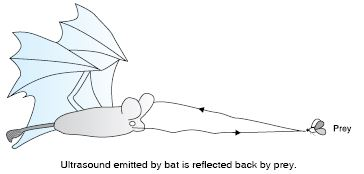##### Question 35:

How is ultrasound used for cleaning?

Ultrasounds are used to clean parts located in hard-to-reach places e.g., complicated electronic components, spiral or odd shaped parts. Appliances to be cleaned are placed in cleaning solutions and ultrasonic waves are sent through cleaning solution. Due to high frequency of ultrasounds, the dust, oil, grease and dirt get detached. The object, thus, gets thoroughly cleaned.

##### Question 36:

Explain the working and applications of a SONAR.

SONAR stands for Sound Navigation and Ranging. It is a device using ultrasonic waves to measure the distance, direction and speed of objects underwater.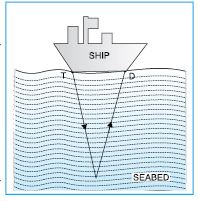SONAR consists of a transmitter T and a detector D, installed below a ship as shown in Fig. The wave produced by transmitter travel through water and is reflected by the seabed. Reflected waves are sensed by the detector, which then converts the ultrasonic waves into electric signals. These signals are interpreted by the detector.
If time interval between transmission and reception is t and speed of sound in sea-water is v, then 2d = vt or d = vt/2, where d is the depth of the sea. This method is also called echo ranging. It also used to locate underwater submarines, icebergs, sunken ship and underwater hills etc.

##### Question 37:

A SONAR device on a submarine sends out a signal and receives an echo 5 s later. Calculate the speed of sound in water if the distance of the object from submarine is 3,625 m.

d = 3,625 m; t = 5s; v = ?
vt = 2d

v =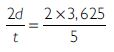v = 1,450 ms-1

##### Question 38:

Explain how defects in a metal block can be detected using ultrasound.

Ultrasounds can be used to detect minor cracks or flaws in metal blocks. Ultrasound is allowed to pass through the metal blocks and detectors are used to detect the transmitted waves. If there is a crack in the metal block, the ultrasound gets reflected back indicating the presence of the cracks or flaws in the metal block.

##### Question 39:

Explain how the human ear works.